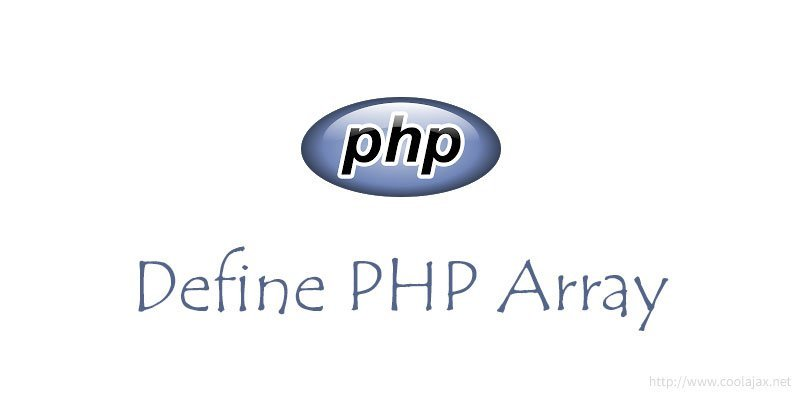# 5 ways to define array in phpArrays are the kings of advanced data structures in PHP. PHP arrays are extremely flexible—they allow numeric, auto-incremented keys, alphanumeric keys or a mix of both, and are capable of storing practically any value, including other arrays.

All arrays are ordered collections of items, called elements. Each element has a value and is identified by a key that is unique to the array it belongs to. As I mentioned in the previous line, keys can be either integer numbers or strings of arbitrary length. So, lets have a look 5 ways to define array in php.

#### – Define an empty array.

`\$my_array_1 = array();`

#### – Define an auto incremented indexed array.

`\$my_array_2 = array("elem_1","elem_2","elem_3");`

#### – Define an array with assigned index(number)

```\$my_array_3 = array(
1=>"elem_1",
2=>"elem_2",
3=>"elem_3"
);```

#### – Define an array with assigned index(string)

```\$my_array_4 = array(
"name"=>"my_name",
"age"=>20,
"sex" =>"male"
);```

#### – Define a multidimensional array:

```\$multi_dim_array =  array();
\$multi_dim_array[] = array("elem_1","elem_2");
\$multi_dim_array[] = array("elem_3","elem_4");
echo \$multi_dim_array;
echo \$multi_dim_array;```